# Monthly Archives: April 2018

## Digging Deeper into … Number Sentences, Equations and Variables (3rd – 6th)

In the Primary Mathematics Curriculum (1999), this topic appears as three separate strand units, all within the strand of Algebra:

• Number Sentences (3rd & 4th class)
• Equations (5th & 6th class)
• Variables (6th class)

However, since these concepts are intrinsically connected, in Operation Maths they are taught in a cohesive and progressive way through third to sixth class.

• Number sentences are mathematical sentences written using numerals (e.g. 1, 5, 67, 809, 1.45, 1/2  etc.) and mathematical symbols (e.g. +, -, x, ÷, <, >, =).
• They include both equations (see below) and inequalities (64 < 82, 23 > -16), although the term inequalities is not specifically used.
• The unknown or missing value in a number sentence (i.e. a variable) can be represented by a frame (box), by a shape, or by a letter, although it should be noted that the Primary Mathematics Curriculum (1999) specifies a preference for a frame (box), up to the introduction of variables in 6th class
• An Equation is a special type of number sentence, containing an equals sign, to show that two expressions are equal (e.g. 5 = 3 + 2, 5 + 6 = 20 – 9, etc.)
• A variable is a value in an expression that can  change or vary. However, when there is only one variable in an equation then the value of that variable can be calculated e.g. a + 6 = 9, 20 = 4b.

Thus, while these strand units are only being formally introduced from third class on, the children have actually been exposed to number sentences, equations and variables (i.e. the frame) since the infant classes.

### Equations

(aka Number sentences with an equals sign)

Understanding equations necessitate the appreciation of the correct meaning of the equals symbol. Many children incorrectly translate the equals symbol (=) as meaning ‘and the answer is…’. This incorrectly reinforces that both its purpose and position is to precede the answer in any calculation, a misconception also reinforced by calculators, where you press the = button to get the answer. Such misunderstanding is
evident when you see responses like these:
5 + 6 =  + 3 , i.e. ‘5 + 6 is 11’
5 + 6 =  + 3 , i.e. ‘5 + 6 + 3 is 14’
Adults may also unwittingly compound this, by using ‘makes’ or ‘gives’ as a synonym for equals.

It is vital that the children recognise that the equals symbol indicates that both sides of the equation (which will be referred to simply as a number sentence until fifth class, when the term “equation” is introduced), are equal to one another/are the same value/are balanced. In this way an actual balance (pan or bucket) and cubes can be extremely valuable to model (and solve) equations e.g. in the images below, the first balance shows that 5 equals a group of 3 and a group of 2, and the second balance shows that 12 equals 3 groups of 4.From Operation Maths 4From Operation Maths 5

Furthermore, teachers should reinforce the correct meaning for the symbol = by only translating it as ‘equals’, ‘is equal to’ and/or ‘is the same as’.

### Inequalities

(aka Number sentences with greater than/less than sign)

Despite the fact that the children have been using the greater than and less than symbols since 2nd Class, many still have difficulties reading them and interpreting their meaning. Using a balance and concrete materials, in a similar way as when teaching equations, can greatly help children to gain deeper understanding of the symbols and their meanings.From Operation Maths 4

Through exploration they can identify what is the maximum number of cubes they can put on a side that is less than the other side, before it makes the balance tip in the other direction, thereby invalidating the number sentence; or the minimum number of cubes they can put on a side that is greater than the opposite side, so as to keep the number sentence true.

### Using estimation strategies

Often, when having to indicate if a given number sentence is true or false, it is not always necessary for the children to calculate both sides of the number sentence exactly. There is (usually) only one true or correct option, meaning that every other answer is incorrect or false. Encourage the children to use their estimation and number sense skills to quickly recognise when a statement is obviously false, e.g. a big difference in the size of numbers on one side versus the other.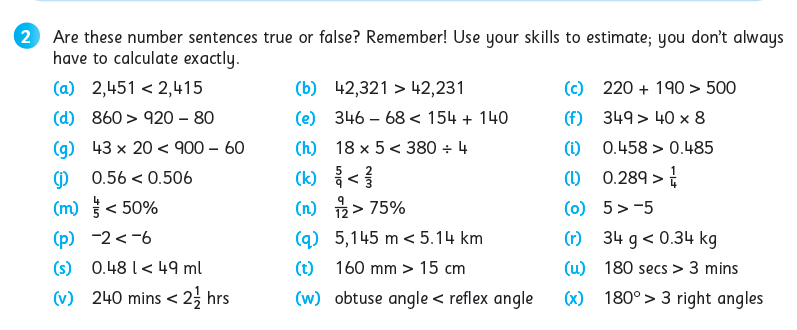While some might view this as a type of ‘cheat’ strategy, in truth, it is more about identifying the most efficient approach, while also reinforcing the value of estimation in general and, particularly, as a way to make calculations easier.

### Translating number sentences into word problems and vice-versa

As mentioned earlier, this is in fact a skill that the children would have been exposed to, and been using, since the infant classes. Furthermore, as this topic is deliberately positioned towards the end of the yearly plans in Operation Maths 3-6, the children will have already been using this skill very regularly in the number, data and measures chapters, prior to this point of the school year.

The curriculum specifies that the children should be enabled to translate number sentences into word problems, both of which can be viewed as abstract representations. Worth noting, is that the curriculum doesn’t emphasise the importance of the translating the number sentences and word problems into concrete and/or pictorial representations. Whereas, in Operation Maths, (in keeping with its overarching CPA approach) , there is significant emphasis placed also on utilising various concrete materials and visual strategies to represent the word problems and number sentences.From Operation Maths 5

The development of visual strategies for problem-solving,  is a central focus of the work throughout the Number chapters. Thus, this topic allows the teacher to revise the visual strategies covered so far and assess how well the children understand them and can apply them.

The interconnectedness of real-life scenarios and mathematical sentences/equations should also be emphasised. At primary level, there should always be some relatable context for any number sentence.
For many children, when looking at a number sentence, it can be difficult to appreciate how a collection of digits and symbols could relate to a real-life scenario with which they can identify. That is essentially what a word problem is; it provides a real-life context within which to frame the numbers and operators involved. Emphasise to the children throughout this topic how the number sentences could be given a real-life story (i.e. word problem), and encourage them to come up with possible stories either verbally or written down.

And, depending on the context given to a particular story, the visual representation may also be different, even though the number sentence/equation may stay the same. For example for the number sentence 7 – 4 = ? the word problem (context) could be either of the following:

• Áine has 7 cookies. Abdul has 4 cookies. How many more cookies has Áine than Abdul?Image created using Bar Modelling eManipulative, accessible on edcolearning.ie

And while the number sentences are the same, both the contexts and the pictorial representations (e.g. bar models, as shown above) are different, as they represent different types of subtraction. In the case of the first word problem, this is describing subtraction as deduction, and a part-whole bar model is more suitable. In the case of the second word problem, this is describing subtraction as difference, and a comparison bar model is more suitable.

### Identifying operation phrases

When the children are translating word problems into number sentences, it is very important that they can understand the context being described and are able to identify that phrases that indicate the operation(s) required.

Regularly interspersed throughout the operations chapters in the Discovery books for Operation Maths 3-6,  there are activities which enable the children to identify and colour-code the specific vocabulary that an indicate the required operation (see example below). This topic provides an ideal opportunity to review this skill and assess/re-teach the children accordingly.From Operation Maths 4, Discovery Book

In particular, many of the Talk Time activities, require the children to suggest ways to verbalise the various equations, e.g:

• The difference between 46 and 18 is equal to the product of 4 and 7; true or false?
• 18 subtracted from 46 equals 4 times 7; true or false?

Where possible the children should suggest alternative phrases for the same equation thus reinforcing the use of correct mathematical language.

### Input and Outputs

In Operation Maths 4 & 5, activities based on inputs and outputs are included as a means to consolidate the children’s understanding of number sentences and their ability to write number sentences (see below).  Input-output activities can provide great scope for problem solving, as well as preparing the children for calculations involving variables in sixth class.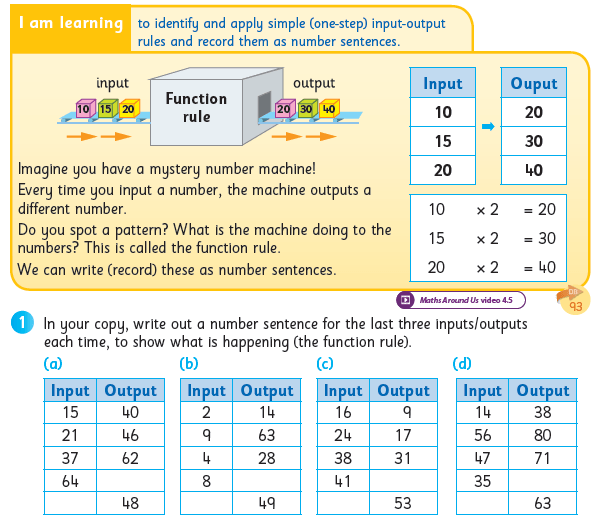From Operation Maths 4

### Variables

Variables are formally introduced in sixth class, although the children have encountered variables (as a symbol or shape to represent a missing value) since they first encountered the frame (answer box).

When calculating with variables, both part-whole bar models and comparison bar models can be very useful to represent the relationship between the known and unknown values.From Operation Maths 6

## Digging Deeper into … Capacity (all classes)

Category : Uncategorized

For practical suggestions for families, and helpful links to digital resources, to support children learning about the topic of capacity, please check out the following post: Dear Family, your Operation Maths Guide to Capacity

Strictly speaking, capacity is the amount (or measure) of a substance (which can be solid, liquid or gas) that something can hold (i.e. a container). That said, in primary mathematics we tend to use capacity as a measure of liquids only (ie not solids or gases), both to avoid confusion and since the children would most commonly see examples of liquids measured using the standard units of capacity (ie litres and millilitres).

### Initial exploration – CPA approach

Like the topics of Length and Weight, and in keeping with the over-arching CPA approach of Operation Maths, children’s initial experiences of capacity at every class level should focus on hands-on activities, using appropriate concrete materials.

In the younger classes, this should occur through exploration, discussion, and use of appropriate vocabulary eg full, nearly full, empty, holds more, holds less, holds as much as/the same as etc. The children should also be enabled to sort, compare and order containers according to capacity.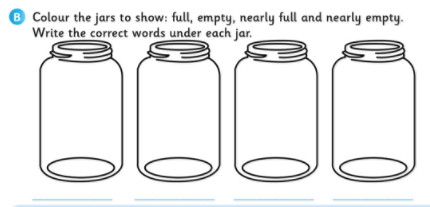From Operation Maths 1

Irrespective of the class level, introductory exploration in this topic could follow the following progression or similar:

• The children examine pairs of empty containers and make comparisons, so as to identify, from sight, which holds more/less. Use questioning to encourage them to assess all available information:
• Which container is wider/narrower?
• Which container is taller/shorter?
• Elicit from the children how they might verify their estimates. Introduce a non-standard measure (e.g. egg-cup, yogurt container, plastic cap from an aerosol, tea/table spoons, plastic syringe, flask etc) and demonstrate how to measure the capacity of a container using a non-standard measure eg (using egg-cup as standard measure):
• Fill an egg-cup with water. Pour this into the target container to be measured. Repeat until container is full and then record the number of egg-cups required.
• OR fill the container with water. From this, pour out an egg-cup full, which is then poured out into a third container (eg basin, plastic box). Repeat until the target container is empty and then record the number of egg-cups that were filled from it.
• OR fill the target container with water. Pour this into a larger container and record the level of the water by marking the level on the side. Pour out the water out into a third container (eg basin, plastic box) to be used as a water store/reservoir. Repeat with other containers to be measured and use the marking on the side of the measuring container to identify which container held the most/least etc. Please note though, that while this method can be used to identify which container holds the most/least, it will not provide a measure of the capacity as a quantity of  non-standard units (unless of course the measuring container has existing markings for litres and/or millilitres)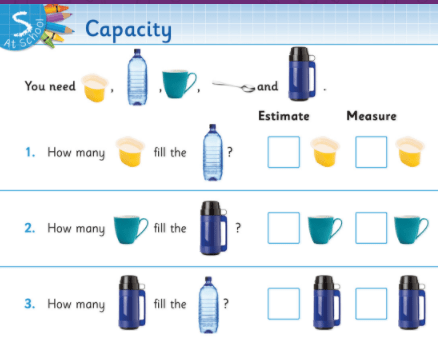From Operation Maths 1

HINT: In order to be avoid unnecessary water wastage and/or a very wet classroom (!), it can be a good idea to conduct the capacity activities outside and over a number of plastic basins/boxes. These can be used to catch spills and to hold the water which can be re-used repeatedly to measure the capacity of the various containers. 20 ml or 50 ml plastic syringes can also be very useful; they are easy for smaller hands to use draw up water and squirt it into a container. And instead of counting ml, ask the children just to record the capacity of the container as the number (count) of syringes that it can hold.

Move on to pairs of containers whose difference in capacity may not be obvious because of the shape and dimension of the containers. Thus, it is important to use a selection of containers that vary in height and width.

This can then progress to incorporate a direct comparison of the capacity of three or more containers. It is important at this stage that the children realise that if A holds more than B and B holds more than C, then, without further direct comparisons, we know that A holds more than C, that A holds the most of all three and C holds the least. This is a very important concept for the children to grasp.

HINT: Use brainstorming to elicit the names of various liquids and container types with which the children are familiar. Use the list to make up an odd one out game, as outlined belowFrom Operation Maths 2 TRB (similar activity also in Operation Maths 1 TRB)

• In a similar way, the children can estimate and record the capacity of containers of objects using standard units (i.e. litres and millilitres; the latter is introduced in third class). Initially, when using the standard unit of a litre (starting from first class) the children will be recording the capacity of containers as being able to hold more than/less than/the same as a litre.

HINT: In 2nd class & 3rd class the children will be using 1/2 litre and 1/4 litre (as opposed to millilitres). This will necessitate using bottles etc that are marked in 1/4 litre intervals. Challenge the children in these classes up to come up with ways to measure and mark these intervals, without having to use millilitres or some type of commercial graduated measure (eg a jug). This task could be given as an alternative homework activity.

When finding the capacity of a container, it is important also to highlight to the children that it is not necessary to fill it to the brim. Show them an example of an unopened litre bottle of water – the height of the water in the
bottle is not to the brim, yet the label shows it contains 1 litre. Thus, the children will develop an understanding that the actual capacity of containers are typically greater than the indicated capacity of the liquid it contains.

Problem Solving: How many are needed to fill? It takes 4 of container A to fill container B. It takes 2 of container B to fill container C. How many of container A are needed to fill C? This can be a very difficult concept to grasp for many children. Some suggestions include using multiples of the real containers to show the relationships between each and drawing pictorial representations using bar models, one of the three key visual strategies for problem-solving used throughout Operation Maths, (shown below).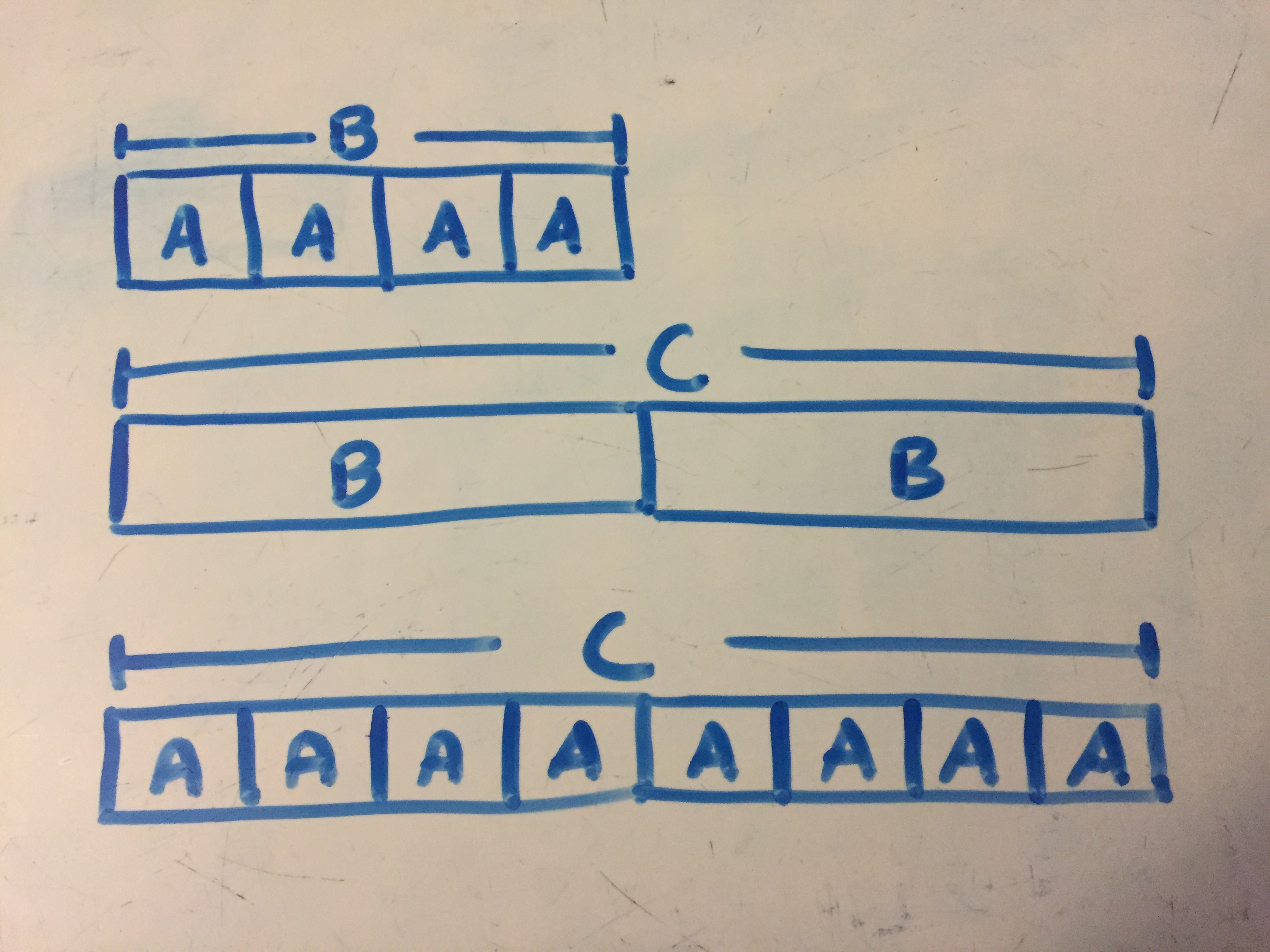### Using more accurate measures

As the children progress in their understanding of the concept of capacity they will begin to appreciate the need for more accurate means to record it; both using smaller standard units (ie millilites) and using measures/containers which are already calibrated/graduated with markings. It is an advantage to have a wide selection of different types of measuring instruments available (including plastic jugs, syringes, measuring spoons, graduated cylinders etc) so that the children appreciate that different measuring instruments are more suitable for certain tasks. When measuring, advise the children also to read the level of liquid at eye level to obtain a more accurate reading.

HINT: Some jugs etc can be purchased relatively cheaply from value shops. Alternatively, ask the children to bring in measuring jugs, containers etc., from home to use in class while working on this topic.

As always, the children should be encouraged to estimate before measuring.  And, rather than estimating the capacity of A, B, C and D before measuring A, B, C and D, it would be better if the children estimated the capacity of A and then measured the capacity of A, estimated the capacity of B and then measured the capacity of B and so on. Thus, they can reflect on the reasonableness of their original estimate each time and use this to refine their next estimate so that it might be more accurate. This helps them internalise a sense of capacity, and to use this sense to produce more accurate estimates.

When the children have experienced using a variety of instruments for measuring capacity, they should then be afforded the opportunity to choose which instrument (and which standard unit) is most appropriate to measure the capacity of various containers. In this way, the children start developing the notion that while many approaches can be taken, some are more efficient than others, and the most efficient approach will also depend on the target object being measured. This is the same as the Operation Maths approach to operations throughout the classes; there can be many approaches and some are more efficient than others, depending on the numbers/operations involved.  The aim is for the children to become accurate, efficient and flexible thinkers.

### Renaming units of capacity

From fourth class on, the children will be expected to rename units of capacity, appropriate to their class level. While changing 1,250 ml to 1 l 250ml or 1.25 l, will typically be done correctly, converting figures which require zero as a placeholder (eg 1 l 50 ml, 2.6 l ) can be more problematic, and can reveal an underlying gap in understanding, that is not revealed by the more obvious measures. In these cases, the children should be encouraged to return to the concrete experiences as a way of checking the reasonableness of their answers, eg:

• “1 l 5o ml…well 1 l  is 1,000 ml and then there’s 50 ml more so it’s 1,000 plus 50, which is 1,050 ml.
• “2.6 l equals 2,600 ml because 1 l is 1,000 ml, so  2 l is 2,000 ml and .6 is slightly more than .5, which is half of a l or 500 ml, which means .6 must be 600 ml”

T-charts, another one of the three key visual strategies for problem-solving used throughout Operation Maths, can be very useful when renaming units of capacity, as can be seen below. These can be partially started on a class board and the children then asked to complete the T-chart with their own choice of capacities as is relevant to the tasks required of them. The children could construct these also to use as a reference, as they progress through this topic.### Capacity & Volume

Volume is introduced officially for the first time in 6th class. It is preferable to introduce the children to volume via cubed units (eg blocks) as opposed to via cubed centimetres (see below).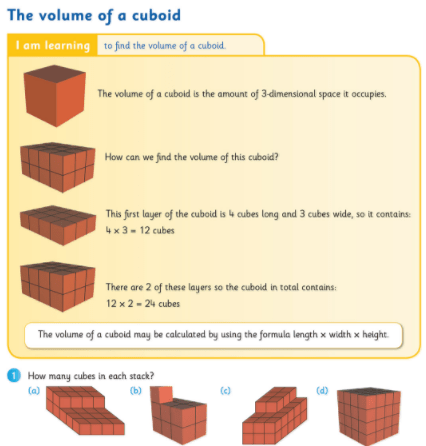From Operation Maths 6

HINT: Did you know that the smallest base-ten blocks (ie those often used as units or thousandths),  are 1 cm cubed? This means that these could be used to build shapes from which the volume of the shapes can be measured and they can be used to measure the approximate volume of an open cuboid eg lunch box, pencil box, etc.

The children may find it challenging to appreciate the relationship between capacity and volume, especially since they may think capacity is exclusive to liquids while volume relates to solids. Providing the children with opportunities to measure the the capacity of a variety of different sized cuboids (eg lunch box) and then measuring its volume using 1 cm cubes, will likely lead the children to discover the connection between the two concepts and that 1cm cubed equals 1 ml.From Operation Maths 6

## Digging Deeper into …. Weight (all classes)

Category : Uncategorized

For practical suggestions for families, and helpful links to digital resources, to support children learning about the topic of weight, please check out the following post: Dear Family, your Operation Maths Guide to Weight

NB: While strictly speaking, the term “mass” is more correct to use than the term “weight” (since mass is measured in kilograms and grams), in Operation Maths, we defer to using the term “weight” as that is the term used in the Primary Maths Curriculum (1999), as well as being the term most frequently used by the general population. To find out more about the difference between mass and weight, click here.

### Initial exploration – CPA approach

Like the topic of Length, and in keeping with the over-arching CPA approach of Operation Maths, children’s initial experiences of Weight at every class level should focus on hands-on activities, using appropriate concrete materials.

In the infant classes, this should occur through exploration, discussion, and use of appropriate vocabulary eg heavy/light, heavier than/lighter than, weighs more/less etc. The children should also be enabled to sort, compare and order objects according to weight.

Irrespective of the class level, introductory exploration in this topic could follow the following progression or similar:

• The children examine pairs of objects and make comparisons, e.g. lunchbox and schoolbag, chair and book, crayon and pencil case. Encourage the children to ‘weigh’ these objects in their hands; using outstretched hands, either to the side or in front of the body, as this can help the children get a better sense of which object is heavier/lighter.
• Elicit from the children how they might verify their hand-weighing. Introduce a balance and demonstrate how to use it. If sufficient balances are available allow one per group of four to six children. If there are not enough commercial balances, a simple alternative is to use a clothes hanger, from which two identical (ask the children why these need to be identical) baskets, trays or bags are hung (see video below).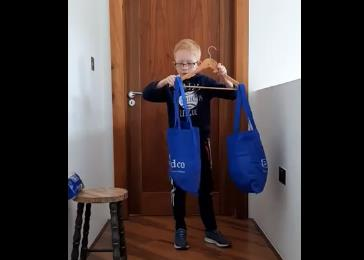• Move on to pairs of objects whose difference in weight may not be obvious, e.g. crayon and marker. Let individual children test pairs of objects on the balance.
• Examine pairs of objects where one is larger but lighter, (e.g. a big piece of paper and a stone, a ball of cotton wool and a pebble, a feather and a marble) and pairs of objects where the objects may have a similar size but different weights (eg a ping pong ball and a golf ball). These experiences enable the children to understand that weight is not related to size.
• This can then progress to incorporate a direct comparison of the weight of three or more objects, to now also include the labels heaviest/lightest. It is important at this stage that the children realise that if A is heavier than B and B is heavier than C, then, without further direct comparisons, we know that A is heavier than C, that A is the heaviest of all three and C is the lightest. This is a very important concept for the children to grasp.
• In a similar way, the children can estimate and record the weight of objects using non-standard units (e.g. cubes, marbles etc) and standard units of weight (e.g. a bag of sugar as a kilogram weight). Initially, when using standard units (e.g. kilogram) they will be recording the weight of objects as being heavier than/lighter than/the same weight as a kilogram.

HINT: 1/2kg and 1/4 kg weights for comparison can be made using that weight of rice, sand etc in ziploc bags. Challenge the children in 2nd class up to come up with ways to make these, and other, weights using only the balance (ie without using a scales). Making these weights could become an alternative homework task.

### Using scales: estimating & measuringFrom Operation Maths 5, Pupils’ Book

As the children progress in their understanding of the concept of weight they will begin to appreciate the need for more accurate means to record weight, i.e. using a weighing scales. It is an advantage to have a wide selection of different types of scales available (including kitchen and bathroom, digital and dial) so that the children appreciate that not all scales are the same, and that their measuring skills have to be flexible enough to be able to adapt to the different types.

HINT: Some scales (eg luggage scales, etc) can often be purchased relatively cheaply from value shops. Alternatively, ask the children to bring in a scales from home to use in class while working on this topic.

As always, the children should be encouraged to estimate before measuring.  This can be done by hand-weighing and can incorporate the comparison of the weight of the unknown object with that of a known weight eg holding a lunch box and a bag of sugar in outstretched hands and estimating the weight of the lunchbox in kg and g based on how much heavier/lighter it feels in comparison to the 1kg weight.

Rather than estimating the weight of A, B, C and D before weighing A, B, C and D, it would be better if the children estimated the weight of A and then measured the weight of A, estimated the weight of B and then measured the weight of B and so on. Thus, they can reflect on the reasonableness of their original estimate each time and use this to refine their next estimate so that it might be more accurate. This helps them internalise a sense of weight, and to use this sense to produce more accurate estimates.

When measuring weight using scales with dials, advise the children to first examine the markings to identify the major makings and to calculate the measure of the minor makings/intervals. When appropriate to the type of scales, encourage the children to read the scales at eye level to obtain a more accurate reading. For demonstrations purposes, a large interactive scales such as the one here, could be used

When the children have experienced using a variety of scales they should then be afforded the opportunity to choose which instrument (and which standard unit) is most appropriate to measure the weight of various items. In this way, they start developing the notion that while many approaches can be taken, some are more efficient than others, and the most efficient approach will also depend on the target object being measured. This is the same as the Operation Maths approach to operations throughout the classes; there can be many approaches and some are more efficient than others, depending on the numbers/operations involved.  The aim is for the children to become accurate, efficient and flexible thinkers.

### Renaming units of weight

From fourth class on, the children will be expected to rename units of weight, appropriate to their class level. While changing 1,250 g to 1kg 250g or 1.25 kg, will typically be done correctly, converting figures which require zero as a placeholder (eg 1 kg 50 g, 2.6 kg ) can be more problematic, and can reveal an underlying gap in understanding, that is not revealed by the more obvious measures. In these cases, the children should be encouraged to return to the concrete experiences as a way of checking the reasonableness of their answers, eg:

• “1kg 50g…well 1 kg  is 1,000g and then there’s 50g more so it’s 1,000 plus 50, which is 1,050g.
• “2.6kg equals 2,600g because 1kg is 1,000g, so  2kg is 2,000 g and .6 is slightly more than .5, which is half of a kg or 500g, which means .6 must be 600g”

T-charts, one of the three key visual strategies for problem-solving used throughout Operation Maths, can be very useful when renaming units of weight, as can be seen below. These can be partially started on a class board and the children then  asked to complete the T-chart with their own choice of weights as is relevant to the tasks required of them. The children could construct these also to use as a reference, as they progress through this topic.